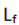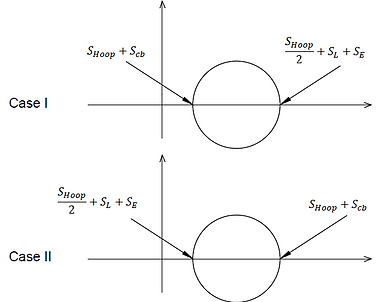top of page

Power boiler code introduces a method for local stress calculation in a lug welded to a boiler tube. This model may be used for verifying created local stresses in a similar lug attaching to a pipe. Using this method requires some cautions. Actually user should know all assumptions used for developing the formula and be careful of its limitations. So in this part, firstly all limitations and assumptions have been mentioned and then the formula and checking methods are clearly described. This method is based on the Ring Model in which a circumferential bending moment developed in the discontinuity area results in a circumferential bending stress as shown in figure 1.

#### Local Stress Calculation in a Lug Based on Power Boiler CodeFigure 1: Circumferential moment and bending stresses in a ring model

1-Assumptions and limitations

• Inch-Pound unit system must be used.

• The method is valid for welded lug attachment.

• The method is based the Ring Model.

• Only in-plane bending moment is considered. The source of this moment comes from an eccentric force not resulting from sustained or expansion loads.

• Shear force is ignored.

2-The checking method description

As early mentioned, the developed local stress is based on the circumferential bending moment. This local stress is proportional to support load and    ratio.Figure 2: Lug model

The code presents tension and compression allowable loads which create circumferential bending allowable stress                           Then the existing load is compared to the proper allowable loads depending on its direction (tension or compression). The allowable loads are function of    , lug angle and allowable stress as follows:(1)

Where K is defined as             and is function of lug angle (θ). Table 6.7 of  can be used to determine K.

has two values depending on the load direction: tension or compression as follows:(2)

(3)

(4)

Where    is pipe basic allowable stress.

It seems expansion stress         and sustained stress       have not been considered in equation (4). So in our opinion,  may be calculated referring to ASME BPVC Section VIII, Div 2, as follows:(5)

Regarding Mohr’s circle shown in figure 3 the intensity stress can be calculated as follows:Figure 3: Mohr’s circles for different possibilities of stresses on an elementThis can be conservatively written as:The circumferential bending allowable stress can be calculated as following:(6)(7)

In our opinion, equations (4), (6) and (7) may be used based on engineer’s judgment, experience and knowledge.

As shown in figure 2-4, if an equivalent system of a support load at point A comprises any in-plane moment, an equivalent added axial force (F*) which creates the same maximum bending stress will be considered as calculated bellow:Figure 4: Eccentric support load on a lug(8)

(9)(10)

So,(11)

If                   , the stress is reduced to its allowable by increasing in b.bottom of page